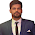# How to Create Equated Monthly Installment (EMI) Calculator Using VBA in Excel

## In this Lesson, we are going to learn how to create EMI calculator using VBA (Virtual Basic for Application) in Excel:

Before creating EMI calculator, we are going to discuss about what is EMI calculator? and what is VBA?.

### EMI Calculator:

EMI calculator is the equated monthly installment calculator which help us to find out how much equal amount you need to pay every month towards repayment of your loan.

### What is VBA in short:

VBA stand for virtual basic for application, is a high class language developed by Microsoft Corporation. You can use this language to develop a program to control Excel spreadsheet.

### What is EMI?

EMI stand for equated monthly installment, a fixed payment amount owed each month on a land / property mortgage or any other loan.

#### Where:

P = Principle loan amount
r  = Annual interest rate / 12
n = Number of monthly installments

above mentioned formula is very complex. But you can use Excel PMT function to calculate the same.

#### PMT function:

=PMT(rate, nper, pv, [fv], [type])

"rate" = Monthly rate (Interest rate / Number of payments per year).

"nper" = Total number of payments (Loan term x Number of payment per year).

"pv" = Present Value (Actual loan amount).

## Steps to create EMI calculator:

By following below mentioned steps you can easily create EMI calculator using VBA in excel:

Step 1: First step is to create the format of EMI calculator in Excel as mentioned below:
Step 2: Now next step is to create a command button by following below mentioned steps:

1. First you need to enable macros in excel by following below steps:
• Click on File tab.
• Click Option.
• Now click on Trust Center, then click on Trust Center Settings.
• In Trust Center, Click on Macro Settings.
• In Macro Settings, tick on Enable all Macros.
• Click OK to save the settings.
2. Now next step to save your workbook as macro enabled workbook as mentioned below:
Now you can see that your file is showing macro sign as mentioned in below snapshot:
3. Now click on Developer tab, click on Insert and select the button as mentioned in below snapshot:
4. Now Draw a button between row 9 and 10 to get the Assign Macro window as mentioned below:
5. Now change the macro name btnCalculateEMI_Click as mentioned in below snapshot:
6. Now Click on New button to get following code window as mentioned below:
7. Now insert the following code as mentioned below:

Dim monthly_rate As Single, loan_amount As Double, number_of_periods As Single, emi As Double
monthly_rate = Range("D8").Value / Range("D7").Value
loan_amount = Range("D5").Value
number_of_periods = Range("D6").Value * Range("D7").Value
emi = WorksheetFunction.Pmt(monthly_rate, number_of_periods, -loan_amount)
Range("D11").Value = emi
8. Now final step is to click on Save button and close this VBA code window as mentioned in below snapshot:
Now you can see that button has been created as mentioned in below snapshot:

Step 3: Now the Next step is to Edit the Button Name by pressing right click on button.
Now you can see that EMI calculator has been ready as mentioned below:
Step 4: Now the final step is to test your EMI calculator by putting values into the calculator and press the button to calculate EMI as mentioned below:
We hope that you will like this lesson.

---Thank You---

1.1.